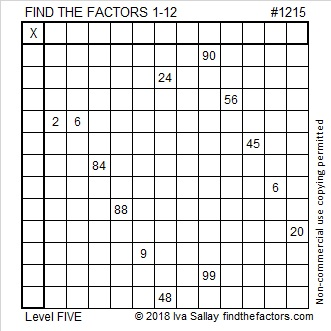# 1215 and Level 5

Level 5 puzzles are a little trickier than level 4 puzzles, but you can still use logic to find the unique solution to today’s puzzle. Stick with it!Print the puzzles or type the solution in this excel file: 12 factors 1211-1220

Here is some information about the number 1215:

• 1215 is a composite number.
• Prime factorization: 1215 = 3 × 3 × 3 × 3 × 3 × 5, which can be written 1215 = 3⁵ × 5
• The exponents in the prime factorization are 5 and 1. Adding one to each and multiplying we get (5 + 1)(1 + 1) = 6 × 2 = 12. Therefore 1215 has exactly 12 factors.
• Factors of 1215: 1, 3, 5, 9, 15, 27, 45, 81, 135, 243, 405, 1215
• Factor pairs: 1215 = 1 × 1215, 3 × 405, 5 × 243, 9 × 135, 15 × 81, or 27 × 45
• Taking the factor pair with the largest square number factor, we get √1215 = (√81)(√15) = 9√15 ≈ 34.856851215 is the hypotenuse of a Pythagorean triple:
729-972-1215 which is (3-4-5) times 243